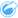# Calculate % Change

When you calculate percent change, you are calculating the change in numerical values over time. Calculating change as a percentage is a form of normalization, which makes it especially useful for comparing areas that are not uniform in size or population.

##### Note:

You can also calculate percent change using the data table.

## Example

A crime analyst is studying the effects of a city's crime reduction strategy. Calculate % Change can be used to determine the effectiveness of the strategy in different districts by using the crime rate before the program started and the crime rate after the program's implementation.

## Use the Calculate % Change capability

Use the following steps to run the Calculate % Change analysis capability:

1. Create a map, chart, or table using the dataset with which you want to calculate percent change.
2. Click the Action button.
3. Do one of the following:
• If your card is a chart or table, click How has it changed? in the Analytics pane.
• If your card is a map, click the Find answers tab and click How is it changed?.
4. Click Calculate % Change.
5. For Choose a layer, select the dataset with which you want to calculate percent change.
6. For Choose number fields, choose the fields you want to use for the initial value and final value. The fields must be a number or rate/ratio.
7. For Name the result field, enter a name for the new field.
8. Click Run.

A new rate/ratio field is added in the chosen dataset.

## Usage notes

Calculate % Change can be found using the Action buttonunder How has it changed? in the Find answers tab. The input layer can be a point, line, or area layer.

Two number fields must be chosen as the initial value and the final value. The number fields will be used in the equation: (final_value-initial_value)/initial_value*100

Calculate % Change adds a new rate/ratio field to the input layer. You must include a new field name to run the calculation.